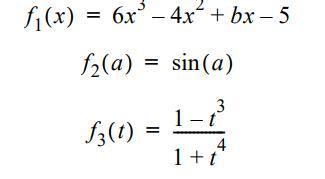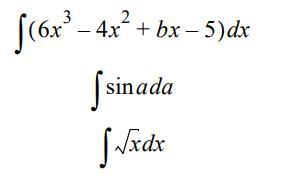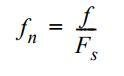# How to Work With Symbolic Toolbox in MATLAB Software Package

Task: get basic knowledge of how to work with Symbolic Toolbox in the MATLAB software package (Part 3).

Solution:

Differentiation

The MATLAB function for performing symbolic differentiation is diff(f,’t’,n) where f is the symbolic expression to differentiate.

– ‘t’ is an optional attribute used to specify the variable of differentiation (there may be more than one symbol present); if not specified, the letter closest to x is used.

– n specifies the order of the derivative, with 1 being the default if n is omitted.

Example:syms x a b t
f1 = 6*x^3-4*x^2+b*x-5;
f2 = sin(a);
f3 = (1 – t^3)/ (1 + t^4);
diff(f1) ans = 18*x^2-8*x+b
diff(f1,2)

ans = 36*x-8

diff(f1,b)
ans = x

diff(f2)

ans = cos(a)

diff(f2,x)

ans = 0 % Expected since not a function of x

diff(f3)

ans = -3*t^2/(1+t^4)-4*(1-t^3)/(1+t^4)^2*t^3

simplify(diff(f3))

ans = t^2*(-3+t^4-4*t)/(1+t^4)^2

Integration

The MATLAB function for performing symbolic integration is int(f,’t’,a,b) or int(f,’t’,’m’,’n’) where

– f is the symbolic expression to integrate

– ‘t’ optionally specifies the variable of integration for the case of several different symbols; if not specified, the letter closes to x is used

– a and b optionally specify numerical limits of integration

– ‘m’ and ‘n’ optionally specify symbolic limits of integration

Example:syms x a b
g1 = 6*x^3 – 4*x^2 + b*x – 5;
g2 = sin(a);
g3 = sqrt(x);
int(g1)

ans = 3/2*x^4-4/3*x^3+1/2*b*x^2-5*x
int(g1,’b’)

ans = 6*x^3*b-4*b*x^2+1/2*b^2*x-5*b
int(g2)

ans = -cos(a)

int(g2,0,pi)

ans = 2

int(g2,0,2*pi)

ans = 0 % Expected for the area under a full cycle
int(g3)

ans = 2/3*x^(3/2)
int(g3,’a’,’b’)

ans = 2/3*b^(3/2)-2/3*a^(3/2)

int(g3,1,2) ans = 4/3*2^(1/2)-2/3

vpa(int(g3,1,2),50) % Variable precision arithmetic

ans = 1.2189514164974600650689182989462641047595625005025Example:

The MATLAB symbolic solution is

syms fn Fs a

Hfs = (1 – a)^2/(1 – 2*a*cos(2*pi*fn) + a^2);

pretty(Hfs)

(1 – a)^2 / 1 – 2 a cos(2 pi fn) + a^2